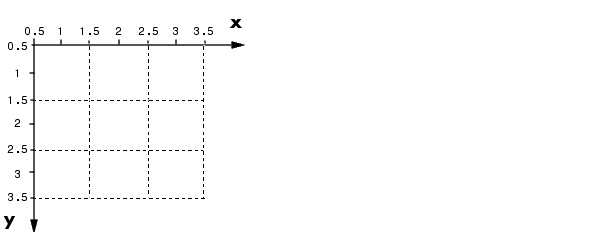Image Processing Toolbox User's GuideSpatial Coordinates

In the pixel coordinate system, a pixel is treated as a discrete unit, uniquely identified by a single coordinate pair, such as (5,2). From this perspective, a location such as (5.3,2.2) is not meaningful.

At times, however, it is useful to think of a pixel as a square patch. From this perspective, a location such as (5.3,2.2) is meaningful, and is distinct from (5,2). In this spatial coordinate system, locations in an image are positions on a plane, and they are described in terms of `x` and `y `(not `r` and `c` as in the pixel coordinate system).

The following figure illustrates the spatial coordinate system used for images. Notice that `y` increases downward.The Spatial Coordinate System

This spatial coordinate system corresponds closely to the pixel coordinate system in many ways. For example, the spatial coordinates of the center point of any pixel are identical to the pixel coordinates for that pixel.

There are some important differences, however. In pixel coordinates, the upper left corner of an image is (1,1), while in spatial coordinates, this location by default is (0.5,0.5). This difference is due to the pixel coordinate system's being discrete, while the spatial coordinate system is continuous. Also, the upper left corner is always (1,1) in pixel coordinates, but you can specify a nondefault origin for the spatial coordinate system. See Using a Nondefault Spatial Coordinate System for more information.

Another potentially confusing difference is largely a matter of convention: the order of the horizontal and vertical components is reversed in the notation for these two systems. As mentioned earlier, pixel coordinates are expressed as (`r`,`c`), while spatial coordinates are expressed as (`x`,`y`). In the reference pages, when the syntax for a function uses `r` and `c`, it refers to the pixel coordinate system. When the syntax uses `x` and `y`, it refers to the spatial coordinate system.

Using a Nondefault Spatial Coordinate System

By default, the spatial coordinates of an image correspond with the pixel coordinates. For example, the center point of the pixel in row 5, column 3 has spatial coordinates `x`=3, `y`=5. (Remember, the order of the coordinates is reversed.) This correspondence simplifies many of the toolbox functions considerably. Several functions primarily work with spatial coordinates rather than pixel coordinates, but as long as you are using the default spatial coordinate system, you can specify locations in pixel coordinates.

In some situations, however, you might want to use a nondefault spatial coordinate system. For example, you could specify that the upper left corner of an image is the point (19.0,7.5), rather than (0.5,0.5). If you call a function that returns coordinates for this image, the coordinates returned will be values in this nondefault spatial coordinate system.

To establish a nondefault spatial coordinate system, you can specify the `XData` and `YData` image properties when you display the image. These properties are two-element vectors that control the range of coordinates spanned by the image. By default, for an image `A`, `XData` is `[1 size(A,2)]`, and `YData` is
`[1 size(A,1)]`.

For example, if `A` is a 100 row by 200 column image, the default `XData` is
`[1 200]`, and the default `YData` is `[1 100]`. The values in these vectors are actually the coordinates for the center points of the first and last pixels (not the pixel edges), so the actual coordinate range spanned is slightly larger; for instance, if `XData` is `[1 200]`, the x-axis range spanned by the image is
`[0.5 200.5]`.

These commands display an image using nondefault `XData` and `YData`.

• ```A = magic(5);
x = [19.5 23.5];
y = [8.0 12.0];
image(A,'XData',x,'YData',y), axis image, colormap(jet(25))```

For information about the syntax variations that specify nondefault spatial coordinates, see the reference page for `imshow`.Pixel Coordinates Displaying and Exploring Images